# Simple Java Graphics

Cay S. Horstmann

I created the Simple Java Graphics library for the CS46A Udacity course. It is similar to the standard Java graphics library, but it works better with BlueJ. Here, you will find an overview of the library, the API documentation, and a cookbook for translating your simple graphics programs to standard graphics.

## Using the Graphics Library

Simply include the classes that you need (or, if you prefer, all classes) in your project. You always need `Shape`, `Canvas`, and `Color`. Then add `Rectangle`, `Ellipse`, `Text`, `Line`, and `Picture` as needed.

Construct a shape and then either `draw` it or `fill` it. (Filling fills the entire shape with its color.)

```Rectangle box = new Rectangle(5, 10, 60, 80);
box.draw();
Ellipse egg = new Ellipse(100, 100, 40, 60);
egg.setColor(Color.YELLOW);
egg.fill();
```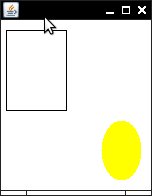You can change a shape by translating (i.e., moving) or growing it.

```box.translate(20, 30); // Move by 20 pixels to the right, 30 pixels down
egg.grow(5, 5); // Grow by 5 pixels on all four sides
```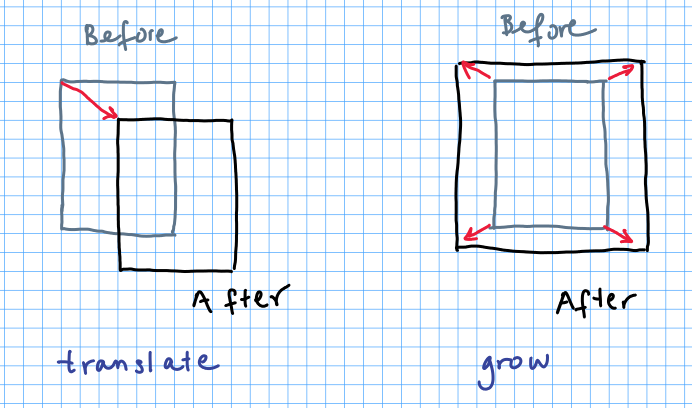All changes to a shape are instantaneous. For example, if you view a `Rectangle` object in BlueJ and change the color, position, or size (with `setColor`, `translate`, or `grow`), the shape gets updated right away.

## Constructing Shapes

To construct a rectangle, give the x- and y- positions of the top left corner, the width, and the height. For an ellipse, give those values for the bounding box of the ellipse.

```Rectangle box = new Rectangle(x, y, width, height);
Ellipse egg = new Ellipse(x, y, width, height);
```For a line, specify the end points. For text, specify the top left corner and the string to be drawn.

```Line segment = new Line(x1, y1, x2, y2);
Text greeting = new Text(x, y, "Hello");
```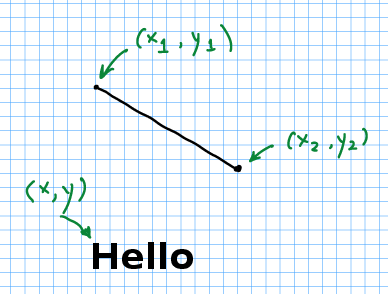To construct a picture, provide the name of the image file, and then move it to the desired location with the `translate` method.

```Picture pic = new Picture("car.png");
pic.translate(x, y);```

You can get the the width and height of any shape with the `getWidth`, and `getHeight` methods. The `getX` and `getY` methods return the coordinates of the top left corner. For convenience, `getMaxX` and `getMaxY` return the coordinates of the bottom right corner.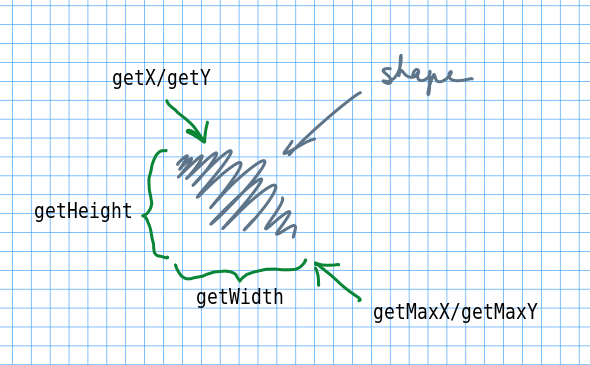## Canvas Methods

All shapes are drawn on an object of type `Canvas`. You don't need to worry about it, but the `Canvas` class has a couple of convenience methods.

`Canvas.pause()` pops up a dialog so that the drawing is stopped until the user dismisses the dialog. This can be useful when you want to see a picture before you change its pixels.

`Canvas.snapshot()` takes a snapshot of the canvas, fades it, and sets the faded image as the canvas background. Any newer drawings appear at full intensity. By taking repeated snapshots, you can show movement.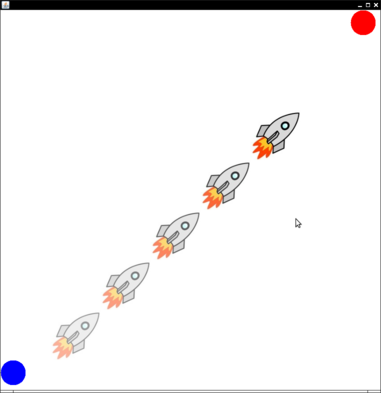## Pictures and Pixels

The `Picture` class can be used to add images to scenes, or to write programs that manipulate pixels.

If you want to do the latter, you can construct an empty picture as

`Picture pic = new Picture(width, height);`

You access the pixels of a picture with the methods `getColorAt` and `setColorAt`. You can use a single integer index to access the pixels.

```Color c = pic.getColorAt(i);
pic.setColorAt(i, Color.RED);```

The index goes from 0 to `pic.pixels() - 1`, where `pic.pixels()` is the total number of pixels in the image. It is the same as `pic.getWidth() * pic.getHeight()`.

This one-dimensional index is useful if you simply want to iterate over all pixels and transform them in a uniform way, for example to make all pixels in an image redder.

Alternatively, you can access pixels by their `x` and `y` coordinates, for example

```Color c = pic.getColorAt(x, y);
pic.setColorAt(x, y, Color.RED);```

Here, `x` goes from 0 to `pic.getWidth() - 1` and `y` goes from 0 to `pic.getHeight() - 1`.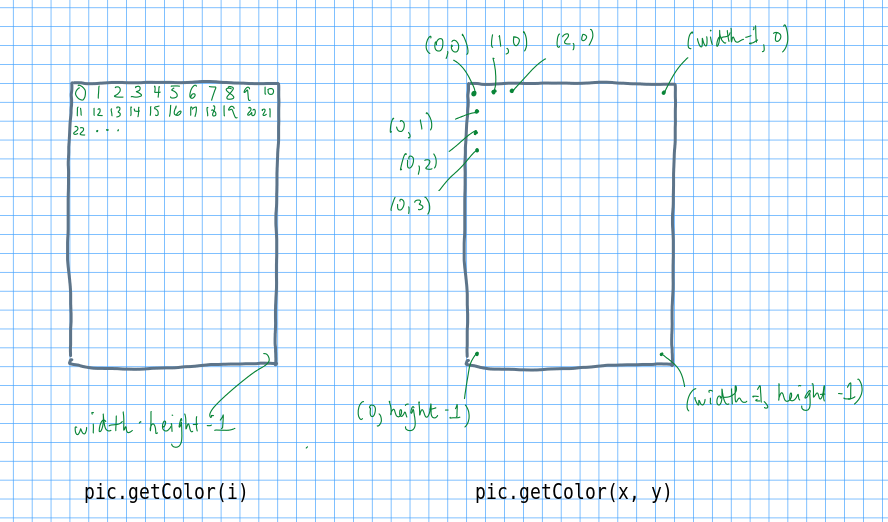Finally, you can get a two-dimensional array of gray levels between 0 and 255 with the call

`int[][] grays = pic.getGrayLevels()`

Why not an array of colors? This method is used for some practice assignments with two-dimensional arrays, and an array of `int` is easier to work with than an array of `Color` values.

Note that the row index is the y-coordinate and the column index is the x-coordinate; that is, `grays[y][x]` is the gray level of `pix.getColor(x, y)`.

## Changing to Standard Java

If you can't use the simple graphics library, make these changes in your program.

• Use `Rectangle2D.Double`, `Ellipse2D.Double`, `Line2D.Double` from the `java.awt.geom` package instead of `Rectangle`, `Ellipse`, `Line`
• Place your drawing code inside a class
```import java.awt.Graphics;
import java.awt.Graphics2D;
import javax.swing.JComponent;

public class MyComponent extends JComponent
{
public void paintComponent(Graphics g)
{
Graphics2D g2 = (Graphics2D) g;
// Your drawing instructions go here
}
}
```
• In the drawing instructions, put the code that you would have placed in `main`, and change all calls `shape.draw()` to `g2.draw(shape)` and `shape.fill()` to `g2.fill(shape)`. However, for `Text` objects, there is no analogous object. Instead, use `g2.drawString(x, y, messageString)`.
• Use `java.awt.Color` instead of the `Color` class. Replace `shape.setColor(c);` with `g2.setColor(c);` just before drawing or filling the shape.
```import javax.swing.JFrame;

public class MyViewer
{
public static void main(String[] args)
{
JFrame frame = new JFrame();
frame.setSize(300, 400); // Change width and height as needed
frame.setDefaultCloseOperation(JFrame.EXIT_ON_CLOSE);
MyComponent component = new MyComponent();